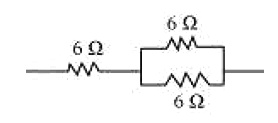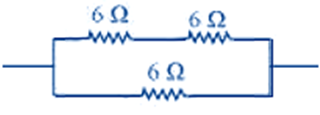3 Tutor System
Starting just at 265/hour

# Show how you would connect three resistors, each of resistance 6 $$\Omega$$, so that the combination has a resistance of (i) 9 $$\Omega$$, (ii) 4 $$\Omega$$.

If we connect the resistors in series, then the equivalent resistance will be the sum of the resistors,

$$\Rightarrow$$ 6 $$\Omega$$ + 6 $$\Omega$$ + 6 $$\Omega$$ = 18 $$\Omega$$,

which is not desired. If we connect the resistors in parallel, then the equivalent resistance will be $$\frac{6}{2}$$ = 3 $$\Omega$$ is also not desired.

Hence, we should either connect the two resistors in series or parallel.

(a) Two resistor in parallelTwo 6 $$\Omega$$ resistors are connected in parallel. Their equivalent resistance will be
$$\frac{1}{(\frac{1}{6} + \frac{1}{6} )}= \frac{6}{2} = 3 \Omega .$$

The third 6 $$\Omega$$ resistor is in series with 3 $$\Omega$$. Hence, the equivalent resistance of the circuit is 6 $$\Omega$$+ 3 $$\Omega$$ = 9 $$\Omega$$.

(b) Two resistor in parallelTwo 6 $$\Omega$$ resistors are in series. Their equivalent resistance will be the sum 6 + 6 = 12 $$\Omega$$.
The third 6 $$\Omega$$ resistor is in parallel with 12 $$\Omega$$. Hence, equivalent resistance will be

$$12 x \frac{6}{12} + 6 = 4 \Omega .$$

Therefore, the total resistance is 4$$\Omega$$